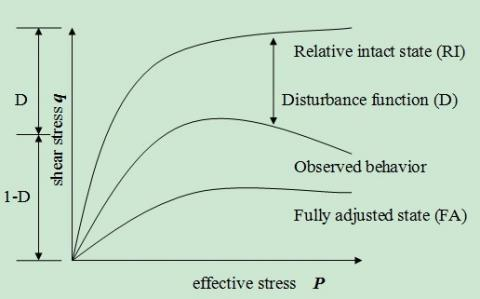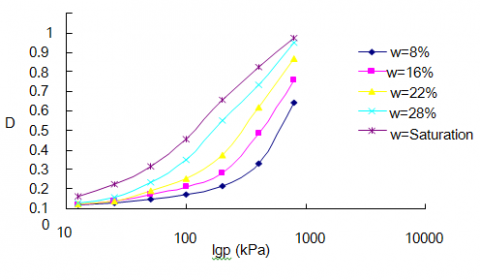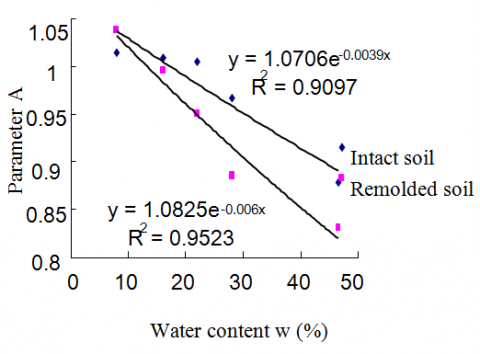# A 1D Compression Model for Loess Based on Disturbed State Concept

A 1D Compression Model for Loess Based on Disturbed State Concept
Department of Architectural Engineering, Hefei University, Hefei 230601, China

Corresponding Author Email:
yantu2000@163.com
Page:
125-129
|
DOI:
https://doi.org/10.18280/rcma.290208
29 January 2019
|
Accepted:
7 April 2019
|
Published:
15 July 2019
| Citation

OPEN ACCESS

Abstract:

Despite being insufficiently compacted, the loess structure can withstand a huge load like the super solid. This paper attempts to disclose how water content affects the bonding and arrangement of loess particles, and how it disturbs the loess structure. For this purpose, five confined compression tests are designed for intact loess and remolded loess of different water contents. Specifically, the disturbance function was defined with void ratio as the parameter based on the disturbed state concept (DSC) theory, considering how the structural features of loess Q3 affects the compressive strength. Next, the evolution law of the disturbance function was explored, and the influence law of water content on the parameters of the disturbance function was investigated. Finally, a DSC-based 1D compression model was constructed for loess Q3. The research results show that the water content has a great impact on the evolution law of the 1D disturbance function and its two parameters, and the influence can be described by exponential function. Besides, the experimental results show that our model can accurately describe how water content affects loess compression.

Keywords:

intact loess, remolded loess, disturbance function, evolution law, disturbed state concept (DSC)

1. Introduction

The geotechnical property differentiates geotechnical materials from other materials. It directly bears on the material structure and inhomogeneity. Different soils have different geotechnical properties, and thus varied structures. The structural difference has become the focus of constitutive modelling in recent years.

The loess is formed through a complex process. The formation spans across the entire quaternary period. The special formation conditions and environment have endowed the loess with special particle properties, such as obvious columnar joints and large pores. Despite being insufficiently compacted, the loess structure can withstand a huge load like the super solid. This unique structure makes it extremely complex to model the mechanical and engineering properties of loess.

Much research has been done on the structure and constitutive modelling of loess. For example, Gao  conducted micro- and meso-scale tests on the microstructure of loess. Shen  put forward a series of constitutive models of loess based on damage mechanics theory. Drawing on soil mechanics theory, Xie  proposed the concept of comprehensive structural potential, and developed constitutive models of loess. Many other scholars [5-10] studied the structure and constitutive models of loess by the disturbed state concept (DSC) theory [11-21].

This paper attempts to disclose how water content affects the bonding and arrangement of loess particles, and how it disturbs the loess structure. For this purpose, five confined compression tests are designed for intact loess and remolded loess of different water contents. Specifically, the disturbance function was defined with void ratio as the parameter based on the DSC, considering how the structural features of loess Q3 affects the compressive strength. Next, the evolution law of the disturbance function was explored, and formulated through curve fitting. On this basis, the influence law of water content on the parameters of the disturbance function was investigated. Finally, a DSC-based 1D compression model was constructed for loess Q3, and proved capable of reflecting how water content affects loess compression.

2. Testing Method

2.1 Sampling

The loess samples were collected from the depth of 5m at some building sites in the northern suburbs of Xi’an, northwestern China’s Shaanxi Province. The samples are drab and in the plastic state, belonging to loess Q3. Each sample was marked on the top and bottom surfaces.

## 1.pngThe granular size of loess is often measured by sieving or with a densimeter. Since the loess Q3 mainly contains silt, with a few clays, the sieving method was adopted for loess grading. The grading curve is shown in Figure 1.

Table 1 lists the main physical-mechanical properties of the loess samples, which were obtained through indoor tests on soil mechanics.

Table 1. The main physical-mechanical properties of the loess samples

 Proportion Water content (%) Natural density (g/cm3) Dry density (g/cm3) Liquid Limit (%) Plastic Limit (%) Plasticity Saturated water content (%) Collapsibility 2.71 22 1.48 1.2 31.3 18.9 12.4 46.5 0.017
3. The DSC Theory

The DSC is a method to simulate the constitutive relation of a material disturbed by stress. Extended from the microstructure change measurement, the DSC does not need to define the microscopic constitutive relation, and excels in simulating the interaction of microcracks.

In the DSC, external forces, whether mechanical, thermal or environmental, can disturb the microstructure of the material. On the microscale, the microstructure will initially enter the unstable state under the disturbance, and gradually shifts towards the stable state; eventually, the microstructure will be stable enough to withstand the external load. On the macroscale, the microstructure changes will inevitably lead to variations in the mechanical properties of the material. In return, the strength change and deformation of the material are evidences to the changes of the microstructure. Therefore, the DSC theory can explain the macroscale mechanical response through microstructure changes, eliminating the need to quantify the change of microstructure.

Under the external load, the microstructure of the material will adjust and reorganize itself from the original relative intact (RI) state to the final fully adjusted (FA) state. This process, accompanied by microcracking or particle movements, can be described by a disturbance function (D). This function describes the disturbance evolution by macro measurement, laying the basis for simulating the constitutive relation of the material. Figure 2 is a sketch map of the DSC.

## 2.jpgFigure 2. The sketch map of the DSC

During the transition from RI state to FA state, the material is considered a mixture of RI part and FA part, both of which are randomly distributed. The RI state can be described with an elastic or an elastoplastic constitutive model. In this state, the material can bear the elastic stress and strain as a continuum. The FA state can be simulated by the critical state model or any other suitable model. In this state, the crack propagation causes damages to the material, and eventually leads to material failure.

4. DSC-Based 1D Compression Model

4.1 Basic hypothesis

The DSC-based 1D compression model was set up based on the following hypotheses:

Hypothesis 1: The intact loess is in the RI state, and the remolded loess in the FA state.

Hypothesis 2: The elastic deformation of the intact loess is the same with that of the remolded loess before yielding, i.e. the elastic deformation has nothing to do with loess structure.

Hypothesis 3: The deformation is elastic before the stress reaches the yield stress.

4.2 Model construction

Based on the DSC, the material deformation is the sum of the deformations in the RI and FA states:

$\varepsilon _{_{ij}}^{a}=(1-{{D}_{\varepsilon }})\varepsilon _{ij}^{i}+{{D}_{\varepsilon }}\varepsilon _{ij}^{c}$    (1)

where εa ij is the observed strain tensor; εi ij is the strain tensor in the RI state; εc ij is the strain tensor in the FA state; Dε is the disturbance function.

Since vi=v0-kilnp, the volume strain in the RI state can be derived from the Modified Cam-Clay Model (MCC):

$\varepsilon _{v}^{i}=\frac{{{k}^{i}}\ln {{p}^{'}}}{{{v}_{0}}-{{k}^{i}}\ln p}$     (2)

where ki is the expansion index of intact loess; v is the specific volume of intact loess (v=1+e).

Since vc=v0-λlnp, the volume strain in the FA state can be depicted as:

$\varepsilon _{v}^{c}=\frac{\lambda \ln {{p}^{'}}}{{{v}_{0}}-\lambda \ln p}$    (3)

where λ is compression index of remolded loess.

Equation (1) shows that the 1D compression model can be constructed directly based on the volume strain and the disturbance function. Hence, the constitutive modelling is transformed to the construction of the disturbance function.

4.3 Definition of disturbance function

The previous experiments have shown that the intact loess differs greatly from remolded loess in compressibility due to their different mechanical performance under the same load. This difference comes directly from the loess structure. Therefore, the disturbance function should reflect the effects of structure on the resistance to loading stress. Since loess is water-sensitive, the disturbance function needs to disclose how water content affects the structure. In confined compression tests, the compressive deformation can be described by the volumetric change, which is essentially the variation in void ratio. Hence, the disturbance function is defined here with void ratio as the parameter:

$D=\frac{{{e}_{0}}-{{e}_{{}}}}{{{e}_{0}}-{{e}_{c}}}$   (4)

where e0 is the initial void ratio; e is the void ratio of intact loess under load; ec is the void ratio under the ultimate load.

The evolution laws of disturbance function for intact loess and remolded loess can be obtained from equation (4). These laws are displayed in Figures 3 and 4, with w being the water content.

## 3.pngFigure 3. The evolution law of the disturbance function for intact loess

## 4.pngFigure 4. The evolution law of the disturbance function for remolded loess

It can be seen from the two figures that the evolution laws of the above disturbance function are suitable for intact loess and remolded loess:

$D=1-A\exp (-B\lg p)$     (5)

where A and B are the parameters of the disturbance function.

4.4 Effect of water content on the parameters of the disturbance function

The parameters A and B of the disturbance function were obtained through least squares fitting for intact loess and remolded loess, respectively, with different water contents.

Table 2. The fitted values of parameters A and B

 Soil type Water content w (%) Parameters Correlation coefficient A B Intact loess 7 1.0148 0.0089 0.964 15 1.0084 0.0015 0.9934 20 1.0054 0.0017 0.9989 26 0.969 0.0023 0.9957 30 0.8792 0.0028 0.9754 Remolded loess 7 1.0374 0.0015 0.9857 15 0.9960 0.0020 0.9988 20 0.9515 0.0024 0.9866 26 0.9856 0.0027 0.9793 30 0.9317 0.0026 0.9663
As shown in Table 2, the disturbance function exhibited a clear variation law with the increase in water content. The parameters A and B also showed regular changes due to the growth of water content. The influence laws, fitted parameters and correlation coefficients are shown in Figures 5 and 6 below.

## 5.pngFigure 5. Influence of water content on parameter A

## 6.pngFigure 6. Influence of water content on parameter B

Figures 5 and 6 show that the influence of water content on parameter A can be fitted by the exponential function for both intact loess and remolded loess, while that on parameter B can be fitted by the power function for both types of soil:

$A={{a}_{1}}{{e}^{{{b}_{1}}w}}, B={{a}_{2}}{{w}^{{{b}_{2}}}}$  (6)

5. Model Verification

The DSC-based 1D compression model of loess can be constructed based on equations (2)~(5) and the relevant parameters in Table 2:

\begin{align} & \varepsilon _{v}^{a}=(1-D)\varepsilon _{v}^{i}+D\varepsilon _{v}^{c} \\ & =1.0051\exp (-0.0018\lg p)\frac{0.0013\ln p}{2.258-0.0013\ln p} \\ & +(1-1.0051\exp (-0.0018\lg p))\frac{0.2246\ln p}{2.258-0.2246\ln p} \\\end{align}     (7)

The established model involves six parameters. The values of these parameters are listed in Table 3 below.

Table 3. The parameters of the DSC-based 1D compression model

 e ki λ A B 1.258 0.0013 0.2246 1.0051 0.0018
The compressive deformation computed by the model were compared with the experimental values on intact loess. The comparison is displayed in Figure 7 below.

## 7.pngFigure 7. Comparison between computed and experimental results

As shown in Figure 7, the compressive deformation computed by our model agrees well with the experimental results, when the stress was relatively small. With the increase of stress, however, the computed compressive deformation was slightly below the experimental results, especially after the stress surpassed the yield stress of the loess. Overall, the two curves in the figure were basically consistent.

6. Conclusions

In this paper, the disturbance function is defined with void ratio as the parameter based on the DSC, considering how the structural features of loess Q3 affects the compressive strength. Next, the evolution law of the disturbance function was explored, and the influence law of water content on the parameters of the disturbance function was investigated. Finally, a DSC-based 1D compression model was constructed for loess Q3. The research results show that:

(1) The evolution law of the established disturbance function echoes with the exponential function. The fitness of the disturbance function was proved by test results.

(2) The influence of water content on parameter A can be fitted by the exponential function for both intact loess and remolded loess, while that on parameter B can be fitted by the power function for both types of soil.

(3) Experimental results show that the DSC-based 1D compression model for loess Q3 can accurately describe the effect of water content on the compression properties of loess.

Acknowledgment

This work is supported by the natural science fund of the university in An Hui province(NO. KJ2019ZD60; NO. KJ2013B223) and  the excellent young talents foundation project in An Hui province (NO. 2012SQRL195) and the talent introduction in He Fei university (NO. 13RC10)  and the provincial quality engineering projects of the education Department of Anhui Province (NO. 2017zhkt383) and  the undergraduate education quality project in He Fei university (NO. 2018HFMOOC04; NO. 2018HFJC05), the authors appreciate the reviewers.

References

 Gao, G.R. (1980). The classification of microstructure of loess and collapsibility. Scientia Sinica, 24(7): 962-974.

 Shen, Z.J., Hu, Z.Q. (2004). Binary medium model for loess. Journal of Hydraulic Engineering, 34(7): 1-6. https://doi.org/10.3321/j.issn:0559-9350.2003.07.001

 Xie, D.Y., Qi, J.L. (1999). Soil structure characteristics of new approach in research on its quantitative parameter. Chinese Journal of Geotechnical Engineering, 21(6): 651-656.

 Cudny, M. (2003). Simple multi-laminate model for soft soils incorporating structural anisotropy and destructurations, Proceedings of the International Workshop on Geotechnics of Soft Soils: Theory and Practice. Hamburg: A. A. Balkema, 431-444.

 Liu, W.Z., Shi, M.L., Miao, L.C. (2010). Analysis of compressibility of structural soils based on disturbed state concept. Rock and Soils Mechanics, 31(11): 3475-3480.

 Desai, C.S., Toth, J. (1996). Disturbed state constitutive modelling based on stress-strain and non-destructive behaviour. International Journal of Solids and Structures, 33(11): 1619-1650. https://doi.org/10.1016/0020-7683(95)00115-8

 Xu, Y. (2011). Research on the disturbed state constitutive model of the structural loess and application. Xi’an: Xi’an University of Architecture and Technology. https://doi.org/10.7666/d.d244042

 Xu, Y.L. (2018). Experimental Study on one-dimensional disturbed evolution law of Q3 loess. Journal of West Anhui University, 34(5): 110-114. https://doi.org/CNKI:SUN:WXXB.0.2018-05-026

 Chu, F., Shao, S.J. (2018). Experimental study on constitutive model of structural Q3 loess in Long dong area based on concept of disturbance state. Journal of Rock Mechanics and Engineering, 37(9): 2180-2188. https://doi.org/CNKI:SUN:YSLX.0.2018-09-017

 Liu, M.D., Carter, J.P., Desai, C.S., Xu, K.J. (2000). Analysis of the compression of structured soils using the disturbed state concept. International Journal for Numerical and Analytical Methods in Geomechanics, 24(8): 723-735. https://doi.org/10.1002/1096-9853(200007)24:83.0.CO;2-V

 Essa, M.J.K., Desai, C.S. (2017). Dynamic Soil-Pile Interaction Using the DSC Constitutive Model, Indian Geotechnical Journal, 47(2): 137–149. https://doi.org/10.1007/s40098-016-0197-3

 Essa, M.J.K., Desai, C.S. (2014) Application of DSC model for offshore pile foundations. In: Proceeding of the 14th International Conference of International Association for Computer Methods and Recent Advances in Geomechanics, Kyoto, Japan, pp. 22–25.

 Desai, C.S. (2015). Constitutive modeling of materials and contacts using the disturbed state concept: Part 2 – Validations at specimen and boundary value problem levels. Computers and Structures, 146: 234-251. https://doi.org/10.1016/j.compstruc.2014.07.026

 Shao, C., Desai, C.S. (2000). Implementation of DSC model and application for analysis of field pile tests under cyclic loading. International Journal for Numerical and Analytical Methods in Geomechanics 24(6): 601-624. https://doi.org/10.1002/(SICI)1096-9853(200005)24:6<601::AID-NAG85>3.0.CO;2-3

 Akhaveissy, A., Desai, C.S. (2013). FE analysis of RC structures using DSC model with yield surfaces for tension and compression. Computers and Concrete, 11(2): 123-148. https://doi.org/10.12989/cac.2013.11.2.123

 Rocchi, G., Vaciago, G., Fontana, M., Prat, M.D. (2013). Understanding sampling disturbance and behaviour of structured clays through constitutive modelling. Soils Found, 53(2): 315-334. https://doi.org/10.1016/j.sandf.2013.02.011

 Schädlich, B., Schweiger, H.F. (2014). Modelling the shear strength of overconsolidated clays with a Hvorslev surface. Geotechnik, 37(1): 47-56. https://doi.org/10.1002/gete.201300016

 Ouria, A., Desai, C.S., Toufigh, V. (2015) Disturbed state concept-based solution for consolidation of plastic clays under cyclic loading. International Journal of Geomechanics, 15(1): 04014039. https://doi.org/10.1061/(ASCE)GM.1943-5622.0000336

 Prashant, A., Penumadu, D. (2015). Uncoupled dual hardening model for clays considering the effect of over consolidation and intermediate principal stress. Acta Geotech., 10(5): 607–622. https://doi.org/10.1007/s11440-015-0377-9

 Desai, C.S. (2016). Disturbed state concept as unified constitutive modeling approach, Journal of Rock Mechanics and Geotechnical Engineering, 8: 277-293. https://doi.org/10.1016/j.jrmge.2016.01.003

 Fan, R.D., Liu, M., Du, Y.J., Horpibulsuk, S. (2016). Estimating the compression behaviour of metal-rich clays via a Disturbed State Concept (DSC) model. Applied Clay Science, 132-133: 50–58. https://doi.org/10.1016/j.clay.2016.05.014